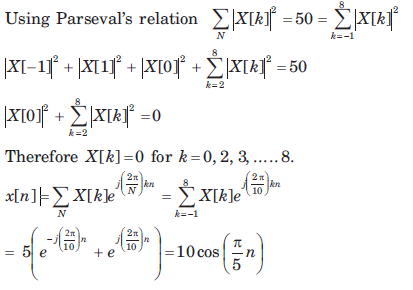Courses

# The Discrete -Time Fourier Series - MCQ Test

## 15 Questions MCQ Test GATE Electrical Engineering (EE) 2022 Mock Test Series | The Discrete -Time Fourier Series - MCQ Test

Description
This mock test of The Discrete -Time Fourier Series - MCQ Test for Electronics and Communication Engineering (ECE) helps you for every Electronics and Communication Engineering (ECE) entrance exam. This contains 15 Multiple Choice Questions for Electronics and Communication Engineering (ECE) The Discrete -Time Fourier Series - MCQ Test (mcq) to study with solutions a complete question bank. The solved questions answers in this The Discrete -Time Fourier Series - MCQ Test quiz give you a good mix of easy questions and tough questions. Electronics and Communication Engineering (ECE) students definitely take this The Discrete -Time Fourier Series - MCQ Test exercise for a better result in the exam. You can find other The Discrete -Time Fourier Series - MCQ Test extra questions, long questions & short questions for Electronics and Communication Engineering (ECE) on EduRev as well by searching above.
QUESTION: 1

### Consider a periodic signal x[n] with period N and FS coefficients X [k]. Determine the FS coefficients Y [k] of the signal y[n] given in question. Que: y[n] = x[ n - no]

Solution: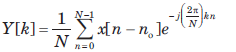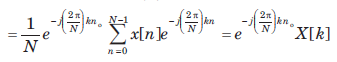QUESTION: 2

### Consider a periodic signal x[n] with period N and FS coefficients X [k]. Determine the FS coefficients Y [k] of the signal y[n] given in question. y[n] = x[n] - x[n-2 ]

Solution: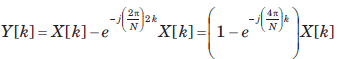QUESTION: 3

### Consider a periodic signal x[n] with period N and FS coefficients X [k]. Determine the FS coefficients Y [k] of the signal y[n] given in question. Que: y[n] =  x[n] + x[n + N/2 ] , (assume that N is even)

Solution:

Note that y[n] =  x[n] + x[n + N/2 ] has a period of N/2 and N has been assumed to be even,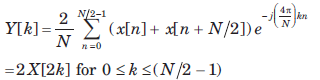QUESTION: 4

Consider a periodic signal x[n] with period N and FS coefficients X [k]. Determine the FS coefficients Y [k] of the signal y[n] given in question.

Que: y[n] = x[n] - x[n + N/2 ] , (assume that N is even)

Solution: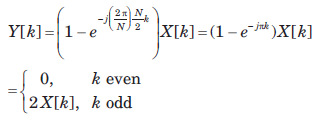QUESTION: 5

Consider a periodic signal x[n] with period N and FS coefficients X [k]. Determine the FS coefficients Y [k] of the signal y[n] given in question.

Que: y[n] = x*[-n]

Solution:

y[n] = x*[-n]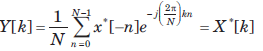QUESTION: 6

Consider a periodic signal x[n] with period N and FS coefficients X [k]. Determine the FS coefficients Y [k] of the signal y[n] given in question.

y[n] = (-1)nx[n], (assume that N is even)

Solution:

With N even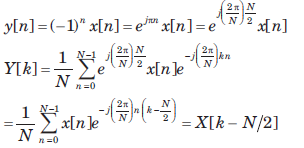QUESTION: 7

Consider a discrete-time periodic signal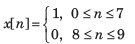with period  N = 10, Also y[n] = x[n] - x[n-1]

Que: The fundamental period of y[n] is

Solution:

y[n] is shown is fig.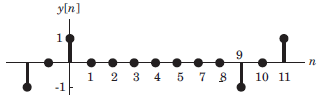It has fundamental period of 10.

QUESTION: 8

Consider a discrete-time periodic signalwith period  N = 10, Also y[n] = x[n] - x[n-1]

Que: The FS coefficients of y[n] are

Solution: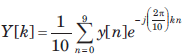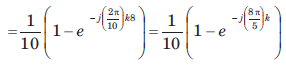QUESTION: 9

Consider a discrete-time periodic signalwith period  N = 10, Also y[n] = x[n] - x[n-1]

The FS coefficients of x[n] are

Solution: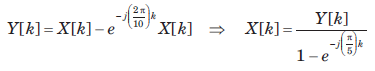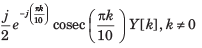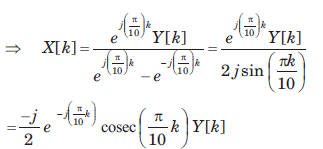QUESTION: 10

Consider a discrete-time signal with Fourier representation.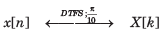In question the FS coefficient Y [k] is given. Determine the corresponding signal y[n] and choose correct option.

Que: Y[k] = X[k-5] + X[k+5]

Solution: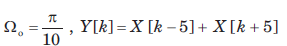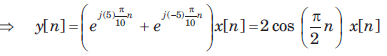QUESTION: 11

onsider a discrete-time signal with Fourier representation.In question the FS coefficient Y [k] is given. Determine the corresponding signal y[n] and choose correct option.

Que: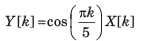Solution: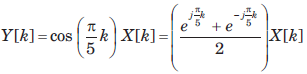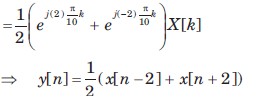QUESTION: 12

Consider a discrete-time signal with Fourier representation.In question the FS coefficient Y [k] is given. Determine the corresponding signal y[n] and choose correct option.

Que: Y[k] = X[k]*X[k]

Solution: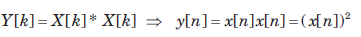QUESTION: 13

Consider a discrete-time signal with Fourier representation.In question the FS coefficient Y [k] is given. Determine the corresponding signal y[n] and choose correct option.

Que: Y[k] = Re{X[k]}

Solution: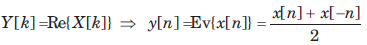QUESTION: 14

A real and odd periodic signal x[n] has fundamental period N = 7 and FS coefficients X [k]. Given that X  = j, X  = 2j, X  = 3j. The values of X ,X [-1], X [-2], and X [ -3] will be

Solution:

Since the FS coefficient repeat every N. Thus

X = X, X = X, X = X
The signal real and odd, the FS coefficient X[k] will be purely imaginary and odd. Therefore X = 0 X[-1] = -X, X[-2] = -X, X[-3]  = -X[-3]
Therefore (D) is correct option.

QUESTION: 15

Consider a signal x[n] with following facts

1. x[n] is a real and even signal

2. The period of x[n] is N = 10

3. X  = 5​ ​ ​ ​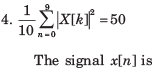Solution:

Since N = 10, X = X = 5
Since x[n] is real and even X[k] is also real and even.
Therefore X = X[-1] = 5.